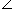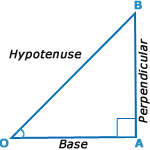## Arithmatical - Heights and Distance

DIRECTIONS : Important Facts and Formulae
1.
Trigonometry:
In a right angled Δ OAB, whereBOA = θ,i . sin θ = Perpendicular / Hypotenuse = AB/OB ii . cos θ = Base / Hypotenuse = OA/OB iii . tan θ = Perpendicular / Base = AB/OA iv . cosec θ = 1/sin θ = OB/AB v . sec θ = 1/cos θ = OB/OA vi . cot θ = 1/tan θ = OA/AB

1.
Trigonometrical Identities:
 i . sin2 θ + cos2θ = 1 ii . 1 + tan2 θ = sec2 θ iii . 1 + cot2 θ = cosec2 θ